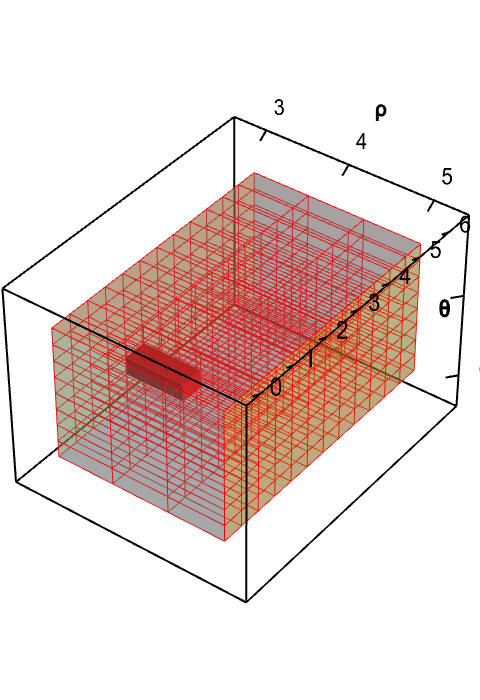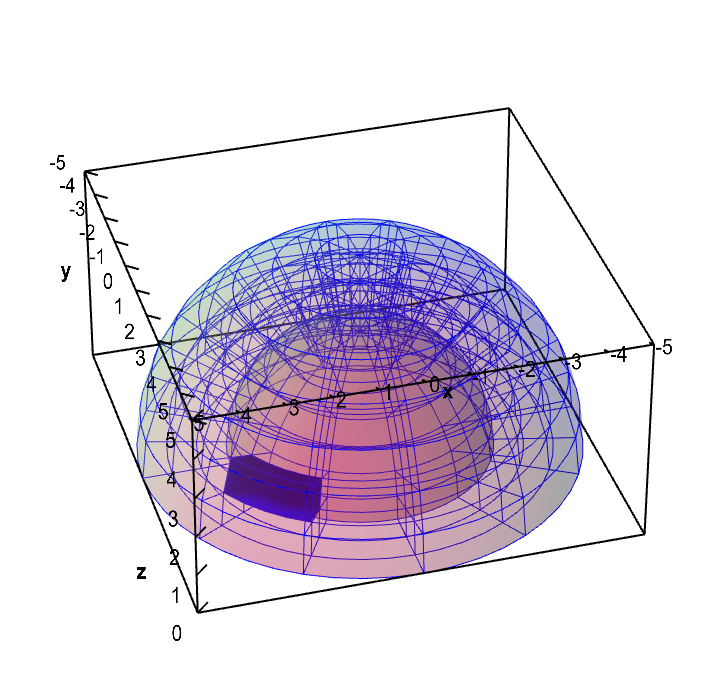# Math Insight

### Applet: A change of variables for a dome-shaped domain changes volumeThe change of variables $\cvarf$ from spherical to Cartesian coordinates maps the rectangular solid $\dlv^*$ (first panel, in red) onto a dome $\dlv$ (second panel, in blue). A point $(\rho,\theta,\phi)$ in $\dlv^*$ in mapped to a point $(x,yz)=\cvarf(\rho,\theta,\phi)$ in $\dlv$. If one chops up $\dlv^*$ into small boxes, the function $\cvarf$ maps the small boxes of $\dlv^*$ onto curvy regions of different size in $\dlv$. You can explore the mapping by dragging the red box $\dlv^*$ or the blue region in $\dlv$. The region in the other panel moves according to the map. The volume of the blue region varies with the position, illustrating how the map $\cvarf$ stretches the region $\dlv^*$ by different amounts depending on the location.